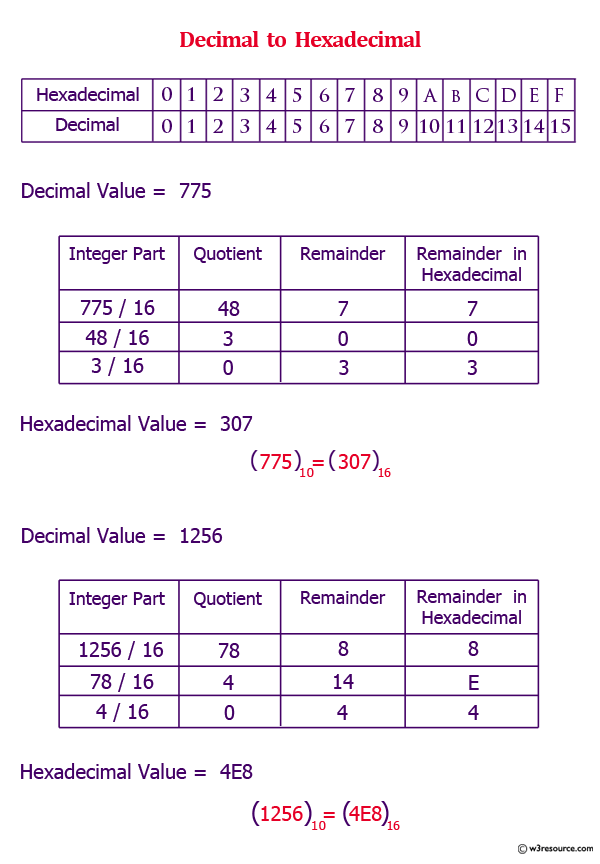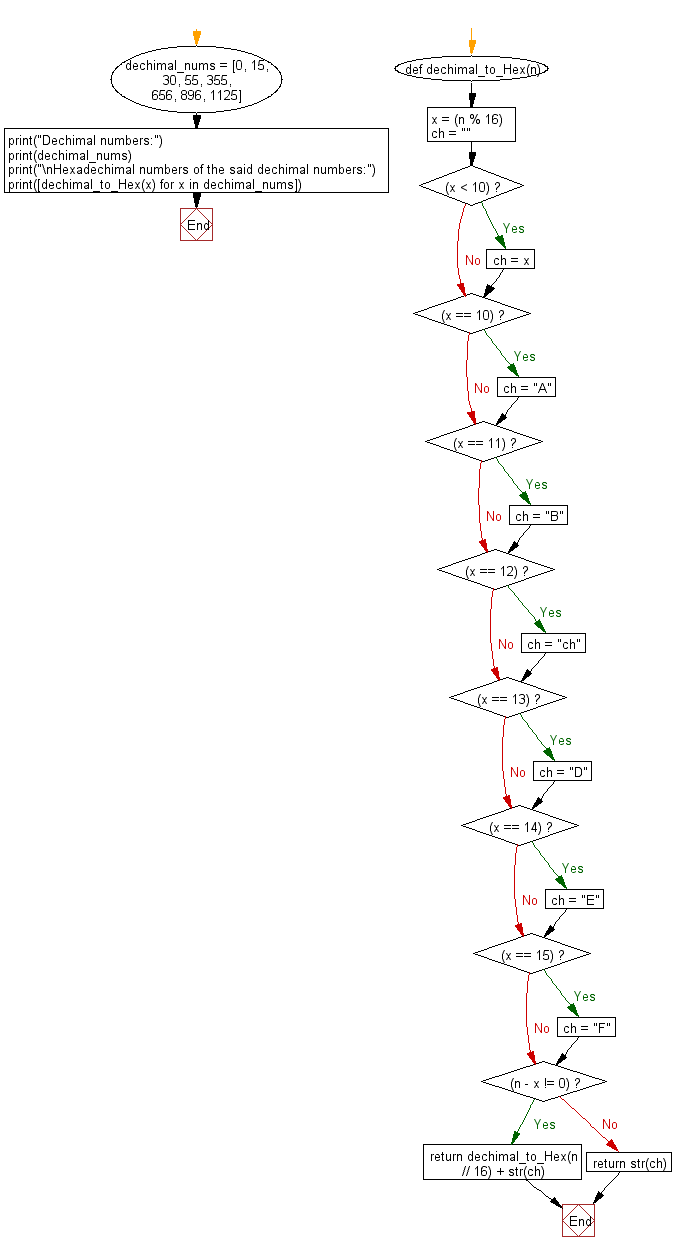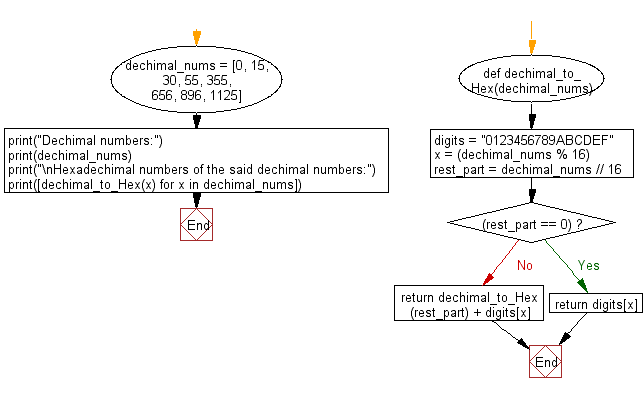﻿ Python: Convert decimal to hexadecimal - w3resource# Python: Convert decimal to hexadecimal

## Python Basic: Exercise-141 with Solution

Write a python program to convert decimal to hexadecimal.

Sample decimal number: 30, 4
Expected output: 1e, 04

Pictorial Presentation:Sample Solution-1:

Python Code:

``````x = 30
print(format(x, '02x'))
x = 4
print(format(x, '02x'))
```
```

Sample Output:

```1e
04
```

## Visualize Python code execution:

The following tool visualize what the computer is doing step-by-step as it executes the said program:

Sample Solution-2:

Python Code:

``````def dechimal_to_Hex(n):
x = (n % 16)
ch = ""
if (x < 10):
ch = x
if (x == 10):
ch = "A"
if (x == 11):
ch = "B"
if (x == 12):
ch = "ch"
if (x == 13):
ch = "D"
if (x == 14):
ch = "E"
if (x == 15):
ch = "F"
if (n - x != 0):
return dechimal_to_Hex(n // 16) + str(ch)
else:
return str(ch)
dechimal_nums = [0, 15, 30, 55, 355, 656, 896, 1125]
print("Dechimal numbers:")
print(dechimal_nums)
print("\nHexadechimal numbers of the said dechimal numbers:")
print([dechimal_to_Hex(x) for x in dechimal_nums])
```
```

Sample Output:

```Dechimal numbers:
[0, 15, 30, 55, 355, 656, 896, 1125]

Hexadechimal numbers of the said dechimal numbers:
['0', 'F', '1E', '37', '163', '290', '380', '465']
```

Flowchart:## Visualize Python code execution:

The following tool visualize what the computer is doing step-by-step as it executes the said program:

Sample Solution-3:

Python Code:

``````def dechimal_to_Hex(dechimal_nums):
digits = "0123456789ABCDEF"
x = (dechimal_nums % 16)
rest_part = dechimal_nums // 16
if (rest_part == 0):
return digits[x]
return dechimal_to_Hex(rest_part) + digits[x]
dechimal_nums = [0, 15, 30, 55, 355, 656, 896, 1125]
print("Dechimal numbers:")
print(dechimal_nums)
print("\nHexadechimal numbers of the said dechimal numbers:")
print([dechimal_to_Hex(x) for x in dechimal_nums])
```
```

Sample Output:

```Dechimal numbers:
[0, 15, 30, 55, 355, 656, 896, 1125]

Hexadechimal numbers of the said dechimal numbers:
['0', 'F', '1E', '37', '163', '290', '380', '465']
```

Flowchart:## Visualize Python code execution:

The following tool visualize what the computer is doing step-by-step as it executes the said program:

Python Code Editor:

Have another way to solve this solution? Contribute your code (and comments) through Disqus.

What is the difficulty level of this exercise?

Test your Python skills with w3resource's quiz

﻿

## Python: Tips of the Day

List comprehension:

```>>> m = [x ** 2 for x in range(5)]
>>> m
[0, 1, 4, 9, 16]
```